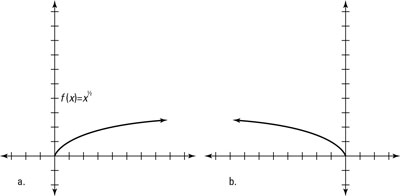##### Trigonometry Workbook For DummiesReflections take a parent function and provide a mirror image of it over either a horizontal or vertical line. You’ll come across two types of reflections:

• A negative number multiplies the whole function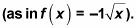The negative outside the function reflects the graph of the function over a horizontal line because it makes the output value negative if it was positive and positive if it was negative. For example, this figure shows the parent function f(x) = x2 and the reflection g(x) = –1x2.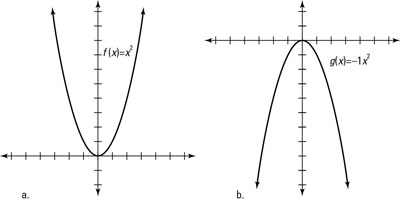If you find the value of both functions at the same number in the domain, you’ll get opposite values in the range. For example, if x = 4, f(4) = 16 and g(4) = –16.

• A negative number multiplies only the input x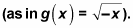The negative inside the function reflects the graph of a function over a vertical line. Vertical reflections work the same as horizontal reflections, except the reflection occurs across a vertical line and reflects from side to side rather than up and down. For this reflection, evaluating opposite inputs in both functions yields the same output. For example, if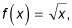you can write its reflection over a vertical line as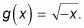When f(4) = 2, g(–4) = 2 as well, as shown in the graph in this figure.